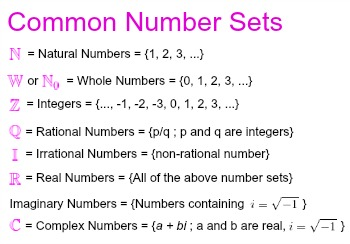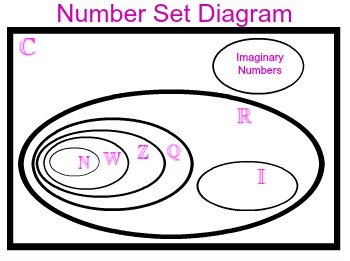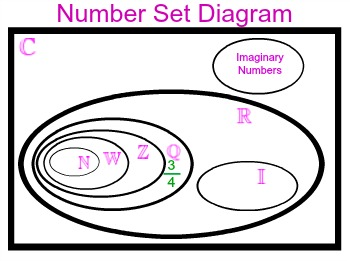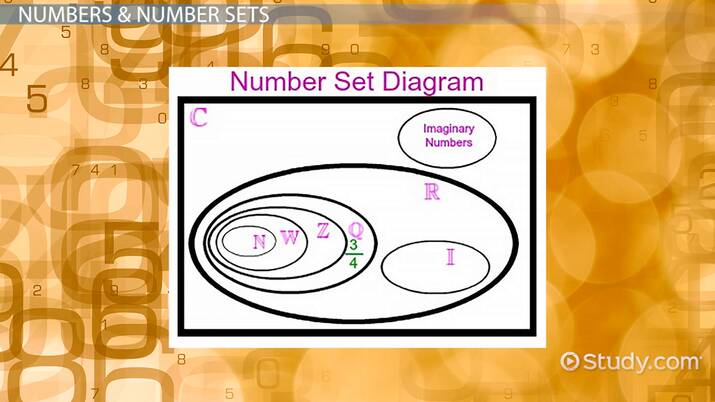# Number Sets: Characteristics & Examples

Lesson Transcript
Instructor: Laura Pennington

Laura received her Master's degree in Pure Mathematics from Michigan State University, and her Bachelor's degree in Mathematics from Grand Valley State University. She has 20 years of experience teaching collegiate mathematics at various institutions.

Number sets are collections of numbers classified as groups according to the values of their elements. Learn about the characteristics of number sets, and explore the common examples of number sets. Updated: 12/21/2021

## Numbers & Number Sets

We all deal with numbers on a daily basis, such as measuring out three-fourths of a cup of cereal for breakfast. They can be classified into number sets, which are just a collection of numbers. The most common number sets, along with the symbols we use to represent each set, are illustrated in the following image:Let's start with the natural numbers, which consist of the counting numbers 1, 2, 3, and so on to infinity; whole numbers include all of the natural numbers and 0. We call them whole numbers because they represent the whole, not a fractional portion of the number. Next, we have the integers, which consist of all of the whole numbers and their negatives.

Rational numbers are those numbers that can be written as a fraction. By comparison, irrational numbers can't be written as a fraction and extend indefinitely after their decimal point without assuming a repeating pattern.

Real numbers are those that we normally work with on a daily basis. They include all of the sets of numbers described, excluding complex numbers and imaginary numbers. Imaginary numbers contain the number i = √-1. Real numbers are complex numbers and can be written as a + bi.

Number sets follow an order where each set is contained in the one that comes after it, with a few exceptions, as shown in the following diagram:A given number can belong to more than one number set. We can place a number in a set if it satisfies the definition of that set. For example, the number 3/4 does not satisfy the definition for a natural, a whole, an irrational, or an imaginary number, or an integer. However, it does satisfy the definitions for rational, real, and complex numbers. Thus, we can place it in our diagram as shown:An error occurred trying to load this video.

Try refreshing the page, or contact customer support.

Coming up next: What is a Mathematical Sequence?

### You're on a roll. Keep up the good work!

Replay
Your next lesson will play in 10 seconds
• 0:04 Numbers & Number Sets
• 1:56 Characteristics of Number Sets
• 3:29 Lesson Summary
Save Save

Want to watch this again later?

Timeline
Autoplay
Autoplay
Speed Speed

## Characteristics of Number Sets

Different number sets are associated with different characteristics. If we know a number lies in a specific set, we know that it has a particular characteristic. This allows us to study numbers and how they work together more easily. Let's explore some of these characteristics.

A closed number set is one where performing a certain operation on two numbers in a set will yield another number in the same set.

For example, adding two natural numbers will yield a natural number, so the natural numbers are closed under addition. However, if we subtracted 7 from 5, we would get 5 - 7 = -2, and -2 is not a natural number. Therefore, natural numbers are not closed under subtraction.

To unlock this lesson you must be a Study.com Member.

### Register to view this lesson

Are you a student or a teacher?